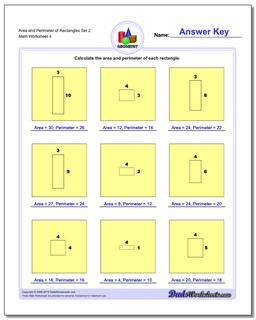PLEASE GO BACK AND USE THE BIG BLUE 'PRINT' BUTTON ON THE PAGE TO PRINT THE WORKSHEET CORRECTLY!Sorry for the trouble! The browser won't print the embedded worksheet PDF directly using the normal 'Print' command in the file menu, so you need to click the big 'Print' button to send just the worksheet and not the surrounding page to the printer.

Math Worksheets: Basic Geometry: Basic Geometry: Area and Perimeter of Rectangles Set 2 (Fourth Worksheet)Area and Perimeter of Rectangles Set 2 (Fourth Worksheet)

PropertyValue
DescriptionArea and Perimeter of Rectangles Set 2: The problems on these geometry worksheets require students to calculate the area and perimeter of rectangles given their dimensions. The worksheets also work great for multiplication practice! (Fourth Worksheet)
Resource TypeWorksheet